# How to Multiply Numbers in Google Sheets? – Multiply Rows, Columns or Cells in Google Sheets

Google Sheets are widely used for two main reasons, namely for saving the data and to perform some mathematical calculations. Multiplication is one of the most important mathematical operators which is widely used in our day-to-day life. With the help of Google Sheets, we can easily perform multiplication such as row multiplication, column multiplication, and so on.

## How to Multiply in Google Sheets?

In Google Sheets, we can perform multiplication calculations using MULTIPLY Function and MULTIPLY Operator which are outlined below in detail.

### MULTIPLY Function In Google Sheets – How to Multiply a Column in Google Sheets?

The steps to perform multiplication calculations using MULTIPLY Function are given below:

• Step 2: Now in Column 1, enter the number 1.
• Step 3: In Column 2, enter the number 2.
• Step 4: Now move to column C and use the formula “=MULTIPLY(A2, B2)”.
• Step 5: Press the “Enter” button and you will see the results as shown below.
• Step 6: To apply the same formula to other cells, drag the formula applied cells to other cells of the data.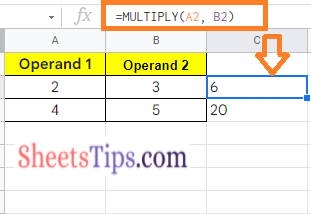### How To do Multiplication in Google Sheets using MULTIPLY Operator?

The steps to perform multiplication using the MULTIPLY Operator in Google Spreadsheets are given below:

• Step 1: Open the Google Sheets to perform multiplication.
• Step 2: Move to Cell A, and enter operand 1 which needs to be multiplied by operand 2.
• Step 3: In Cell B, enter operand 2 which needs to be multiplied by operand 1.
• Step 4: Now move to Cell C and enter the formula “=A2*B2”.
• Step 5: Press the Enter button and you will see the results as shown below.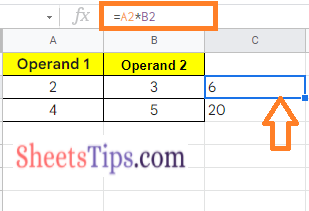### How to Multiply Numbers in Google Sheets?

Let us consider you don’t have any data in Cell A1 and Cell B1, but you simply want to perform some calculations with the help of numbers in your hand. The steps to multiply numbers in Google Sheets are as follows:

• Step 1: Open the Google Spreadsheet to perform the multiplication of numbers.
• Step 2: In this example, I want to Multiply 11 by 11. So we are moving to cell A1. Alternatively, you can move to the desired cell where you want to draw the results.
• Step 3: Now to multiply using MULTIPLY Operator, simply enter the formula =11*11 and press the enter key.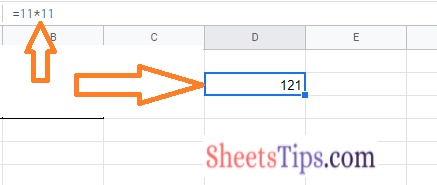Alternatively, you can also multiply 11 by 11 using MULTIPLY Function. To draw the results using the MULTIPLY function, enter the formula =MULTIPLY(11,11).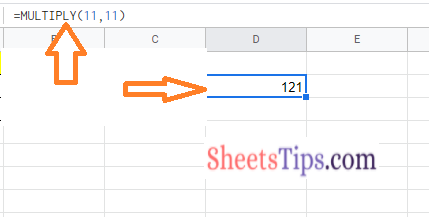### How to Multiply Rows in Google Sheets?

The steps to multiply rows in Google Sheets are as follows:

• Step 1: Open the Google Sheets and enter Operand 1 in Row 1.
• Step 2: Enter the Operand 2 in Row 2.
• Step 3: Now move to Rows 3 and enter the formula “=MULTIPLY(A2,A3)”.
• Step 4: Press the “Enter” key and you will see the results.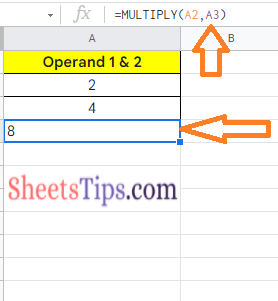Alternatively, you can also use the formula =A2*A3 and press enter key and you will see the results.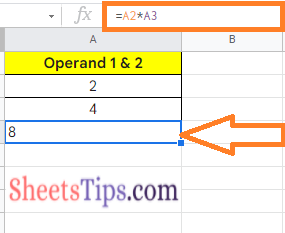### How to Multiply Two Columns in Google Sheets?

While the previous examples focused on multiplying single values or cells, in this part, I will show you how to use a simple formula to multiply two columns (or even more than two columns).

Assume you have the following dataset and wish to calculate the multiplied value of cells in the row in column C.

The formula for doing so is as follows:

=ARRAYFORMULA(A1:A10*B1:B10)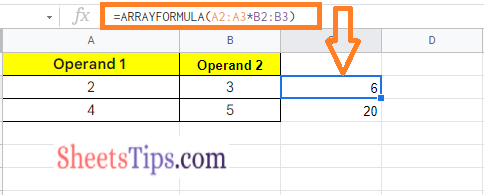Because this is an array formula, you only need to put it in cell C1 and the rest of the column cells will be filled automatically.